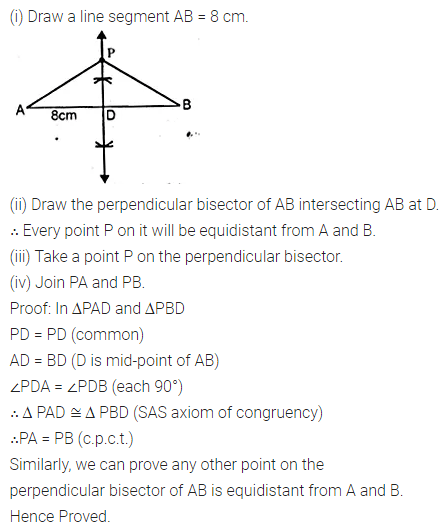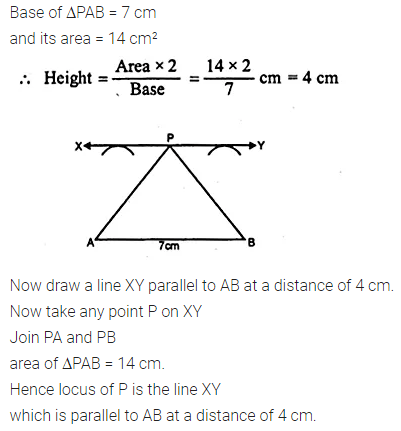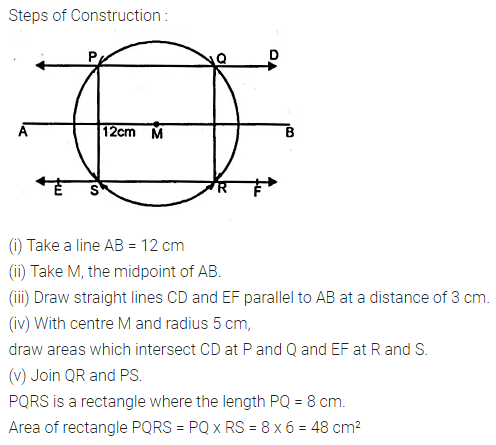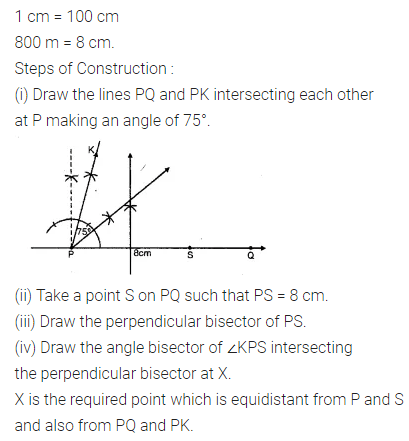# ML Aggarwal Class 10 Solutions for ICSE Maths Chapter 14 Locus Chapter Test

## ML Aggarwal Class 10 Solutions for ICSE Maths Chapter 14 Chapter Test

ML Aggarwal Class 10 Solutions for ICSE Maths Chapter 14 Locus Chapter Test

Question 1.
Draw a straight line AB of length 8 cm. Draw the locus of all points which are equidistant from A and B. Prove your statement.
Solution:Question 2.
A point P is allowed to travel in space. State the locus of P so that it always remains at a constant distance from a fixed point C.
Solution:Question 3.
Draw a line segment AB of length 7 cm. Construct the locus of a point P such that area of triangle PAB is 14 cm².
Solution:Question 4.
Draw a line segment AB of length 12 cm. Mark M, the mid-point of AB. Draw and describe the locus of a point which is
(i) at a distance of 3 cm from AB.
(ii) at a distance of 5 cm from the point M. Mark the points P, Q, R, S which satisfy both the above conditions. What kind of quadrilateral is PQRS? Compute the area of the quadrilateral PQRS.
Solution:Question 5.
AB and CD are two intersecting lines. Find the position of a point which is at a distance of 2 cm from AB and 1.6 cm from CD.
Solution:Question 6.
Two straight lines PQ and PK cross each other at P at an angle of 75°. S is a stone on the road PQ, 800 m from P towards Q. By drawing a figure to scale 1 cm = 100 m, locate the position of a flagstaff X, which is equidistant from P and S, and is also equidistant from the road.
Solution:Question 7.
Construct a rhombus PQRS whose diagonals PR, QS are 8 cm and 6 cm respectively. Find by construction a point X equidistant from PQ, PS and equidistant from R, S. Measure XR.
Solution:Question 8.
Without using set square or protractor, construct the parallelogram ABCD in which AB = 5.1 cm. the diagonal AC = 5.6 cm and the diagonal BD = 7 cm. Locate the point P on DC, which is equidistant from AB and BC.
Solution:Question 9.
By using ruler and compass only, construct a quadrilateral ABCD in which AB = 6.5 cm, AD = 4 cm and ∠DAB = 75°. C is equidistant from the sides AB and AD, also C is equidistant from the points A and B.
Solution:ML Aggarwal Class 10 Solutions for ICSE Maths